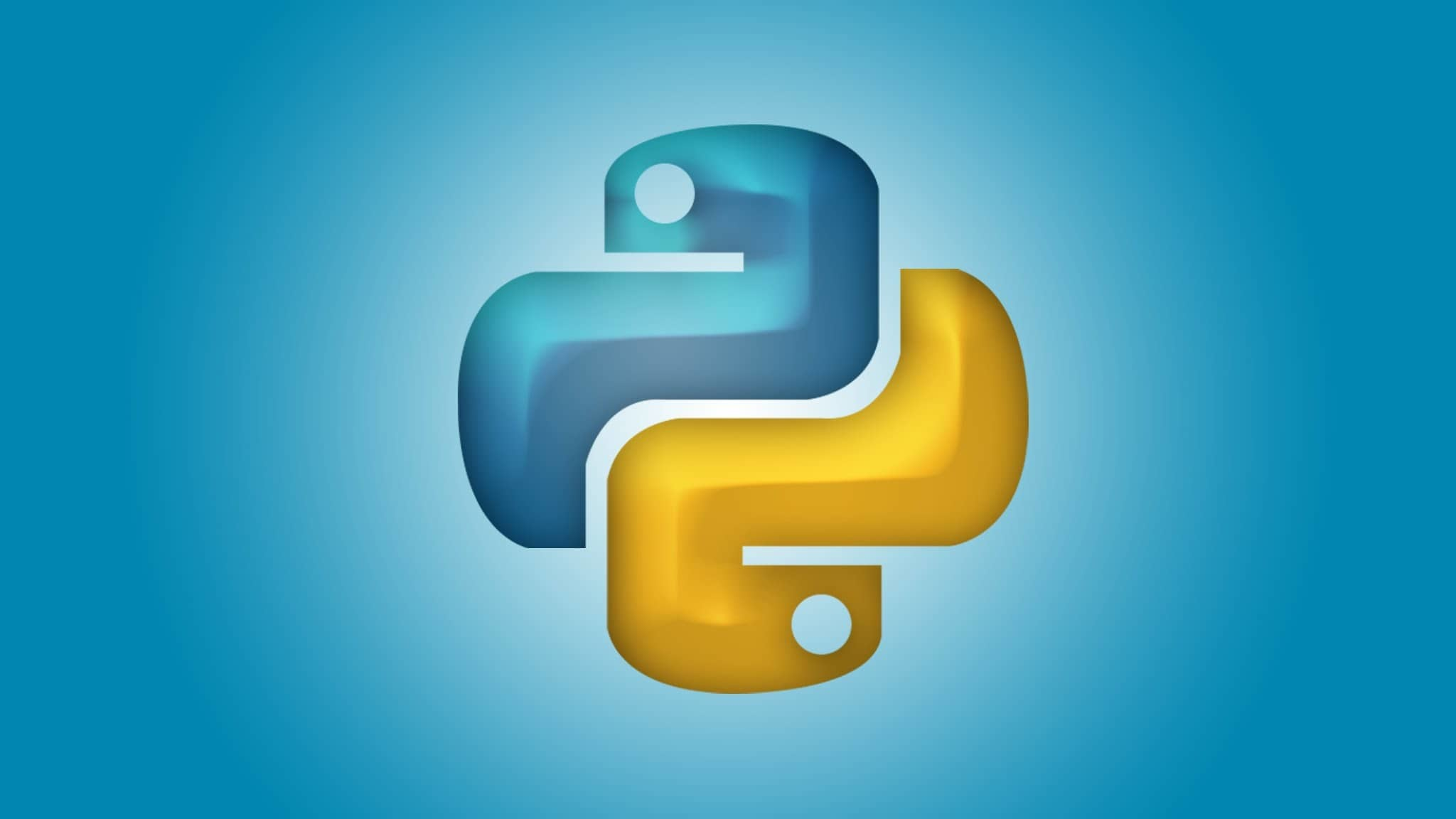October 2, 2023

All technologies, only pure source code

# Bagels a logic game in Python

In Bagels, a deductive logic game in Python, you must guess a secret three-digit number based on clues.This is the first of a list of examplesPython programming language - logo

In Bagels, a deductive logic game in Python, you must guess a secret three-digit number based on clues. The game offers one of the following gints in response to your guess:

• Pico: when your guess has a correct digit in the wrong place
• Fermi: when your guess has a correct digit in the correct place
• Bagels if your guess has no correct digits.

You have 10 tries to guess the secret number.

## How it works

Keep in mind that this program uses not integer values but rather string values that contain numberic digits. For example, `426` is a different value than ‘426’. We need to do this because we are performing string comparison with the secret number, not math operations.

Remember that `0` can be a leading digit: the string ‘026’ is different from ’26’, but the integer ‘026’ is the same as ’26’.

## The code

```import random

# (!) Try setting this to 1 or 10
NUM_DIGITS = 3

# (!) Try setting this to 1 or 10
MAX_GUESSES = 10

def main():
print('''Bagels, a deductive logic game.

I am thinking of a {}-digit number with no repeated digits.
Try to guess what it is. Here are some clues:

When I say:    That means:
Pico         One digit is correct but in the wrong position.
Fermi        One digit is correct and in the right position.
Bagels       No digit is correct.

For example, if the secret number was 248 and your guess was 843,
the clue would be Fermi Pico.'''.format(NUM_DIGITS))

# main game loop
while True:
# This stores the secret number the plaer needs to guess:
secretNum = getSecretNum()

print('I have thought up a number')
print(' You have {} guesses to get it right'.format(MAX_GUESSES))

numGuesses = 1
while numGuesses <= MAX_GUESSES:
guess = ''

# keep looping until they enter a valid guess
while len(guess) != NUM_DIGITS or not guess.isdecimal():
print('GUees #{}: '.format(numGuesses))
guess = input('> ')

clues = getClues(guess, secretNum)
print(clues)

numGuesses += 1

if guess == secretNum:
# There are correct, so break out of this loop
break
if numGuesses > MAX_GUESSES:
print('You have run out of guesses')

# Ask player if they want to play again
print('Do you want to play again? (yes or no)')
if not input('> ').lower().startswith('y'):
break

print('Thanks for playing!')

def getSecretNum():
""" Returns a string made up of NUM_DIGITS unique random digits. """
# create a list of digits 0 to 9
numbers = list('0123456789')
# shuffle them into random order
random.shuffle(numbers)

# get the first NUM_DIGITS digits in the list for the secret number:
secretNum = ''
for i in range(NUM_DIGITS):
secretNum += str(numbers[i])

return secretNum

def getClues(guess, secretNum):
""" Returns a string with the Pico, Fermi, Bagels clues for a guess and secret number pair """
if guess == secretNum:
return 'You got it!'

clues = []

for i in range(len(guess)):
if guess[i] == secretNum[i]:
# A correct digit is in the correct place
clues.append('Fermi')
elif guess[i] in secretNum:
# A correct digit is in the wrong place
clues.append('Pico')
if(len(clues) == 0):
# There are no correct answers
return 'Bagels'
else:
# Sort the clues into alphabetical order so their original order
# doesn't give information way
clues.sort()

# Make a single string from the list of string clues
return ' '.join(clues)

# if the program is run (instead of imported), run the game:
if __name__ == '__main__':
main()
```

## The Help function

Now, in code of Bagels a logic game in Python, you see in line 60 that after the function `getSecretNum` I added 3 quotation marks (`"""`). In Python, you can use triple quotation marks (“””) to create a `docstring` for a function, class, module, or method.

A docstring is a string literal that documents what the code does, how to use it, what parameters it takes, what it returns, and any other information that might be useful for users or developers. This docstring follows the PEP 257 conventions for docstring formatting. You can access the docstring of any Python object by using its `__doc__` attribute or the built-in `help()` function.

So, in my code I added after the `main()` function a call to the `help` for the function `getSecretNum`. In this way, the program prints on screen the help for the function before continues with the main part. In the following screenshot, the result in Visua Studio Code.

This site uses Akismet to reduce spam. Learn how your comment data is processed.